# November 2021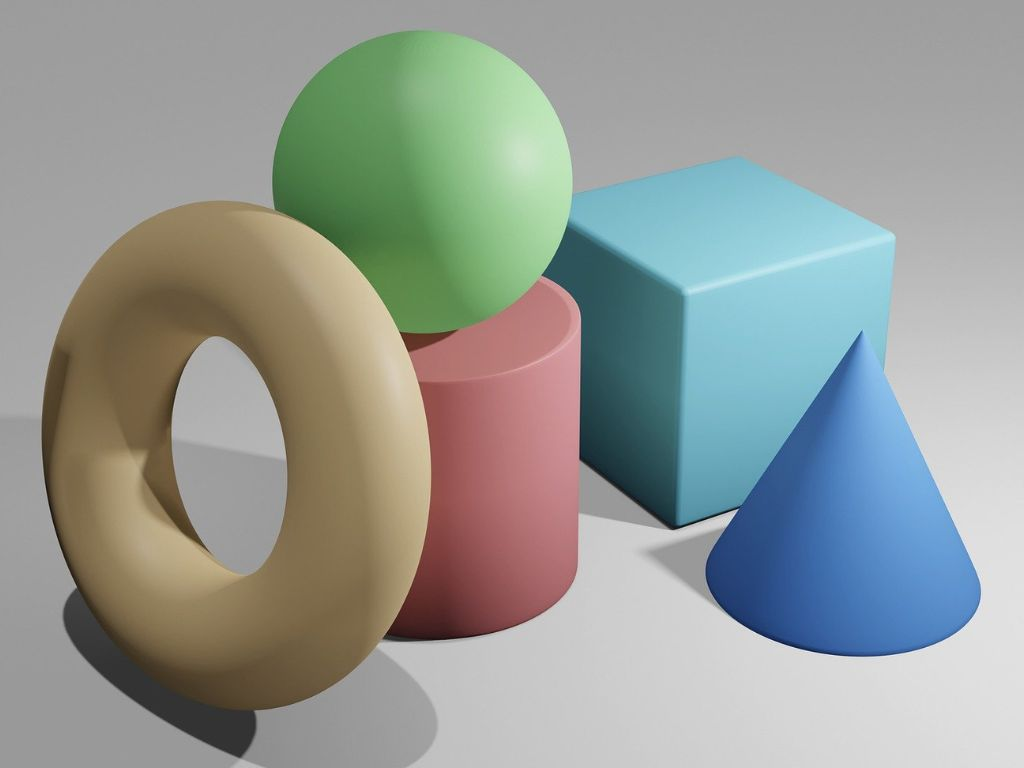## Surface Areas and Volumes Class 10th

Introduction We are familiar with solid shapes or 3D (three-dimensional) shapes like cubes, cuboids, cylinders, cones, spheres, etc. We have studied these shapes and their properties in the previous classes. In class 9th, we studied the basics of surface areas and volumes of solid shapes. We know all the formulae related to surface areas and …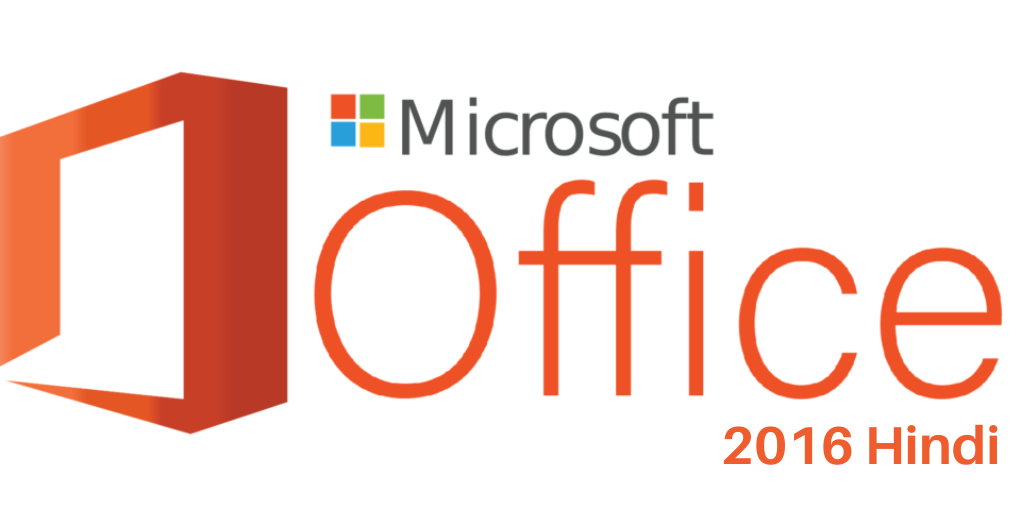## About Microsoft office 2016 Hindi

INTRODUCTION DISCUSSION TOPIC OF MICROSOFT OFFICE SUITES 2016. Brief Introduction of Microsoft Company – माइक्रोसॉफ्ट कंपनी दुनिया की अग्रणी सॉफ्टवेयर के लिए एक प्रसिद्ध कंपनी है, जिसकी विरासत को बढ़ा-चढ़ाकर नहीं बताया जा सकता है। जहां माइक्रोसॉफ्ट कंपनी विश्व स्तर पर कंप्यूटर सॉफ्टवेयर उत्पाद बनाने में दुनिया में नंबर वन है। वर्तमान में, Microsoft विभिन्न …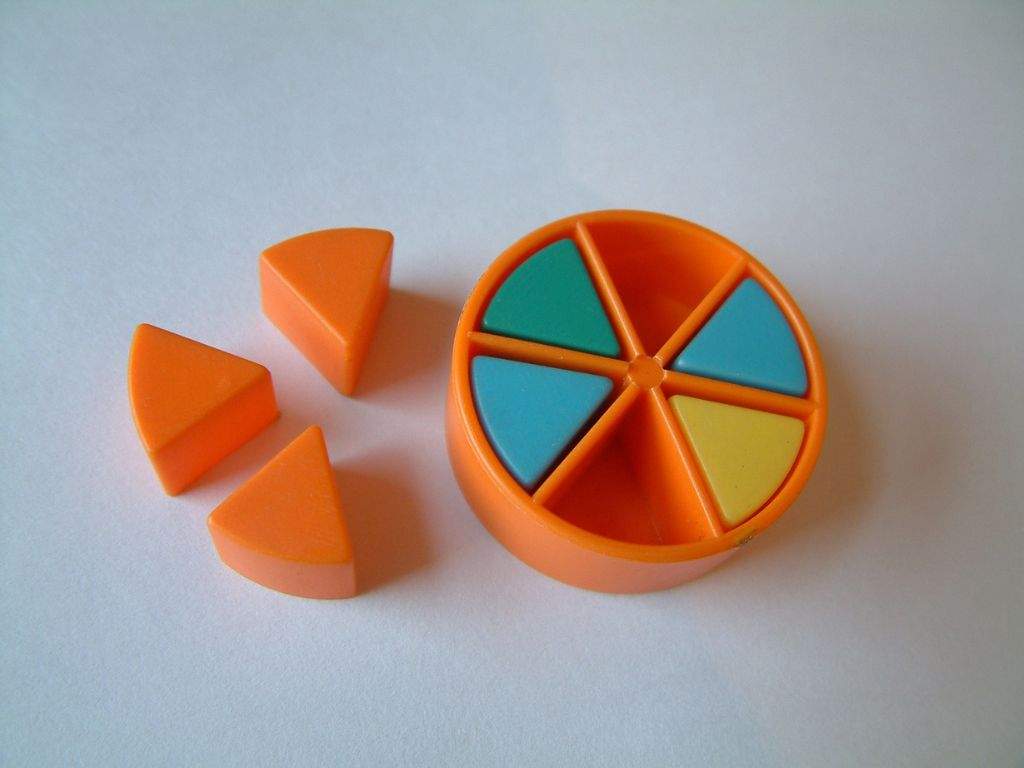## The Similarity of Triangles Class 10th

Introduction The triangle is the shape, we are well known about it and its properties. We have studied different triangles and terms related to them like a scalene triangle, equilateral triangle, isosceles triangle, the area of the triangle, the perimeter of a triangle, the median of a triangle, the altitude of the triangle, etc. In …About Microsoft office 2016 DISCUSSION TOPIC OF MICROSOFT OFFICE SUITES 2016. Brief Introduction of Microsoft Company – Microsoft Company is a well-known company for the world’s leading software, whose legacy cannot be overstated. Where Microsoft company is number one in the world in making computer software products globally. Currently, Microsoft produces a variety of software …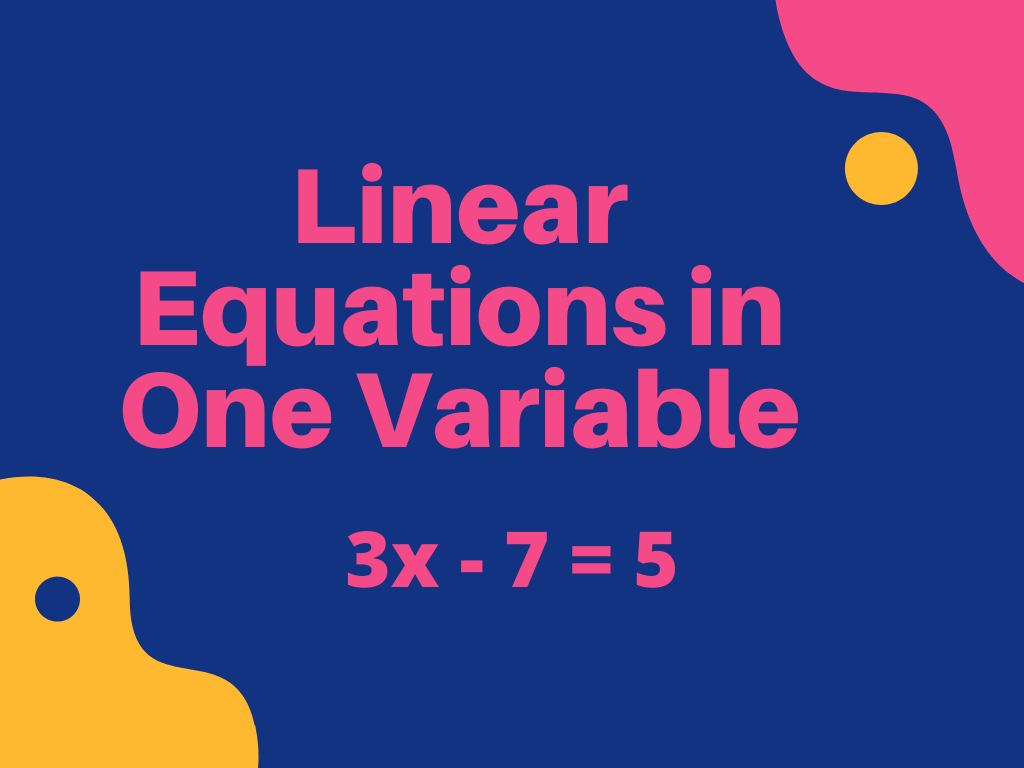## Linear Equations in One Variable Class 8th

Introduction We are familiar with algebraic expressions and equations because we have already studied these in the previous class. Algebraic expressions are mathematical expressions that have a combination of numbers and the English alphabet letters. In the equations, there is the equality sign (=) that is not used in algebraic expressions. Some examples of algebraic …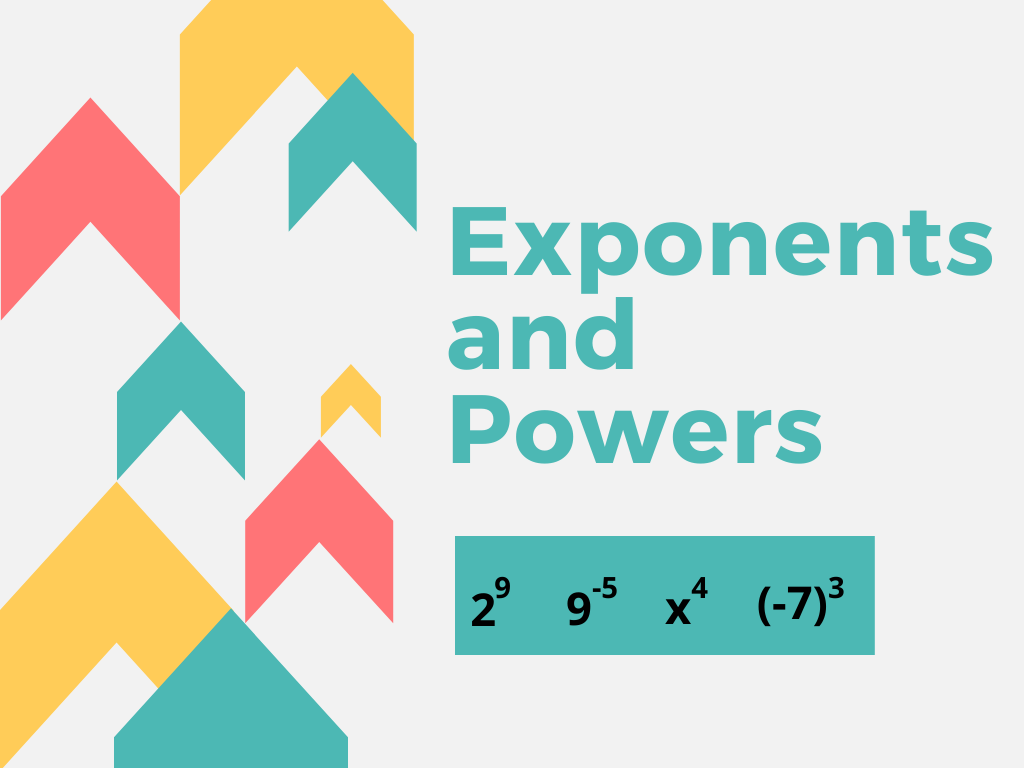## Powers and Exponents Class 8th

Introduction In the previous class, we have already studied powers and exponents. We know that exponents are used to write large numbers in a suitable and convenient form. In large calculations, powers and exponents are very important and useful. In class 8th, we shall explore more about powers and exponents with the help of their …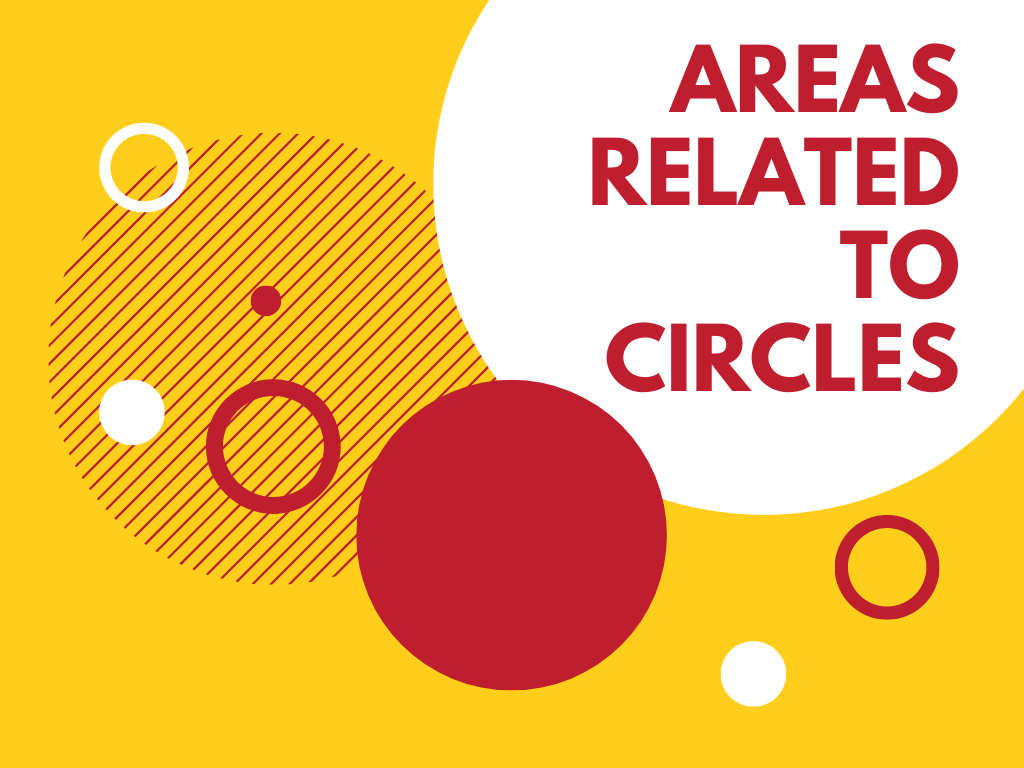## Areas Related to Circles Class 10th

Introduction We have already studied some methods to find the perimeter and area of different types of plane figures like square, rectangle, triangle, circle, trapezium, rhombus, etc. in the previous classes. In our daily life, we see many circular objects like rings, bangles, plates, wheels, roti, etc. All these objects are helpful in studying circular …